# Introduction:

To create a function or to implement the algorithm first we define function as “def”. Also, we can define function by putting parameter inside the function.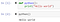Snap-1

To return the output from the function we need to write the “return” statement as given below: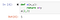Snap-2

There are few advantages of python functions :

a. Function reduces the code length and avoid the same codes again and again

b. Function divides the complex problem into simpler one and makes it understandable

c. Function reduces the chances of errors which makes the code more distinct.

• Filter()
• Recursion()
• Lambda()
• Map()
• Reduce()
• Enumerate()
• Isinstance()

# Filter():

Syntax of filter() method is: filter(function, iterable)

Filter method takes two parameters:

a. Function- Function that is operated on the iterable.

b. Iterable- Iterable which is to be filtered, could be sets, lists, tuples, or containers of any iterators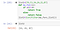Snap-3

# Recursion():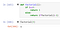Snap-4

In the above example multiply() is a recursive function as it calls itself.

# Lambda():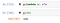Snap-5

In lambda function we can multiple arguments.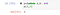Snap-6

The power of lambda is better shown when you use them as an anonymous function inside another function.

# Map():

The returned value from map() (map object) can be passed to functions like list(), set().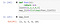Snap-7

# Reduce():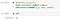Snap-8

We mostly use reduce function when we need any consolidated result or summation result. Here in the above pic, we have imported reduce from “functools” module and then we have executed the code.

# Enumerate():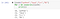Snap-9

# Isinstance():

If the type parameter is a tuple, this function will return True if the object is one of the types in the tuple.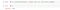Snap-10

Here in the above pic. “Hello” is a string value and it’s present in the type hence it has returned as True.

Data Science Aspirant, Learning Python, SQL, Data Science, ML

## More from Subhradeep Guha

Data Science Aspirant, Learning Python, SQL, Data Science, ML